AP State Syllabus AP Board 6th Class Maths Solutions Chapter 9 2D-3D Shapes Ex 9.1 Textbook Questions and Answers.

## AP State Syllabus 6th Class Maths Solutions 9th Lesson 2D-3D Shapes Ex 9.1Question 1.
What is the name of four sided polygon? Draw the rough sketch.
Solution:
A four sided polygon is called a quadrilateral.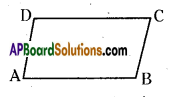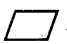ABCD is a quadrilateral.

Question 2.
Draw a rough sketch of pentagon.
Solution:
A five sided polygon is called a pentagon.
So, pentagon has five sided figure.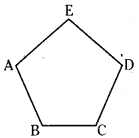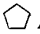ABCDE is a pentagon.Question 3.
Write all the sides of the given polygon ABCDEF.
Solution:
Given polygon ABCDEF is a Hexagon.
Its six sides are $$\overline{\mathrm{AB}}, \overline{\mathrm{BC}}, \overline{\mathrm{CD}}, \overline{\mathrm{DE}}, \overline{\mathrm{EF}} \text { and } \overline{\mathrm{FA}}$$
So, it is called a Hexagon.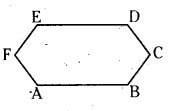Question 4.
Write the interior angles of the polygon PQRST.
Solution:
Given the polygon PQRST has five sides.
So, it is called a Pentagon.
It has five interior angles.
They are ∠TPQ, ∠PQR, ∠QRS, ∠RST and ∠STP.
They can also be written as ∠P, ∠Q, ∠R, ∠S, ∠T.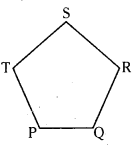Question 5.
Measure the length of the sides of the polygon PQRST.
Solution:
The given polygon has five sides.
They are $$\overline{\mathrm{PQ}}$$ = 2cm; $$\overline{\mathrm{QR}}$$ = 2.5 cm;
$$\overline{\mathrm{RS}}$$ .= 2.4 cm; $$\overline{\mathrm{ST}}$$ = 2.2 cm, $$\overline{\mathrm{PT}}$$ = 2.5 cm
It’s a pentagon.